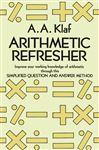ArithmeticHome   Algebra   Geometry   Arithmetic   Trigonometry   Probability/Statistics   Calculus   Mechanics GED   SAT   ACT   College Mathematics   GRE   School Supplies   About Us

Arithmetic

 Electronic Textbooks TDownloads - Preview, Get, Practice!Paperback Textbooks (New and Used)Examples

 Radicals: Simplify √50 + √2 - 2√18 + √8.        50 + √2 - 2√18 + √8 = √(25 x 2) + √2 - 2√(9 x 2) + √(4 x 2)                                     = 5√2 + √2 - (2 x 3)√2 + 2√2                                     = 6√2 - 6√2 + 2√2                                     = 2√2                                         Answer: 2√2 Simple Interest: The sum of \$400.50 is invested at interest rate 12% for 4 years. Find the simple interest for the period and the final amount on the investment.  Simple Interest = Principal x Rate x Time                         = 400.50 x (12/100) x 4                         = \$192.24.  The final amount is the Interest + Principal = 192.24 + 400.50 = \$592.74for Math Practice,  SAT  ACT

 More School Supplies and other useful items *  * *